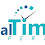# Fibonacci Series :Java Program

package javaPrograms;

import java.util.Scanner;

public class FibonacciSeries
{

public static void main(String arr[])
{
Scanner sc=new Scanner(System.in);
int a=0,b=1;
System.out.println("Enter no. to repesent size of Fibonacci series");
int n=sc.nextInt();

System.out.println("0\n1");

for (int i=0;i<n; i++)
{
int nextNumber=0;
nextNumber=a+b;
System.out.println(nextNumber+" ("+ "a="+a+"  b="+b+")");
a=b;
b=nextNumber;

}

}
}

Output:
--------------------------------------------------------------------------------------------------------------------------

Enter no. to repesent size of Fibonacci series
12
0
1
1 (a=0  b=1)
2 (a=1  b=1)
3 (a=1  b=2)
5 (a=2  b=3)
8 (a=3  b=5)
13 (a=5  b=8)
21 (a=8  b=13)
34 (a=13  b=21)
55 (a=21  b=34)
89 (a=34  b=55)
144 (a=55  b=89)
233 (a=89  b=144)

1.Good Post! Thank you so much for sharing this pretty post, it was so good to read and useful to improve my knowledge as updated one, keep blogging.

Python Training in electronic city

DataScience with Python Training in electronic city

AWS Training in electronic city

Big Data Hadoop Training in electronic city

Devops Training in electronic city

2.Thanks for the informative article. This is one of the best resources I have found in quite some time. Nicely written and great info. I really cannot thank you enough for sharing.DataScience with Python Training in Bangalore

3.This comment has been removed by the author.

Emoji
(y)
:)
:(
hihi
:-)
:D
=D
:-d
;(
;-(
@-)
:P
:o
:>)
(o)
:p
(p)
:-s
(m)
8-)
:-t
:-b
b-(
:-#
=p~
x-)
(k)